# GPL Suspension Information

Introduction:

This will be a four part article that covers what we know about GPL's suspension system. The first part will cover the springs, the second will cover the bump rubbers, the third will cover ride height, and the fourth will cover what this knowledge might imply for setups.

Much of this information was orginally discovered by Gene Fryman. I have independently confirmed Gene's findings and added to them.

Suspension Deflection:

GPL normally uses a suspension travel of 19 centimeters (7.5 inches) from full droop to full bump. However, it may be possible for the suspension to be compressed more than 19 centimeters and the code extrapolates the suspension force beyond the 19 centimeter compression point.

Springs:

A common misconception has been that spring rate and wheel rate (as specified in the setup menu) are different. This is normally true in real life, but in GPL, they are exactly the same as the code does not apply any mechanical advantage from the suspension arms. So when discussing the rates in GPL, wheel rate and spring rate can be used interchangeably. Figure 1 is a picture of a real world shopping cart spring that is a very good depiction of how GPL's wheel and spring work together. This clearly shows a real world suspension where wheel rate is the same as spring rate.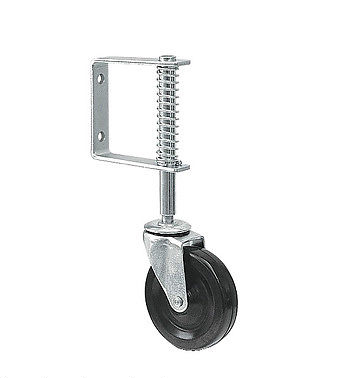GPL simulates non progressive springs that are vertically mounted. The spring uses a constant rate measured in pounds per inch of spring compression. As the spring is compressed, the force it exerts increases at a linear rate. Althought the code slightly tilts the springs for rather obsure reasons, for our purposes we can assume that the springs are always vertical. Again, Figure 1 is good depiction of this.

Figure 2 is a graph that shows the force exerted by a 100 pounds per inch spring as the suspension is compressed. The vertical axis is the spring force in pounds and the bottom axis is the suspension deflection in centimeters. The graph shows the linear increase in spring force that continues in a straight line up to and beyond the 19 centimeter suspension deflection point.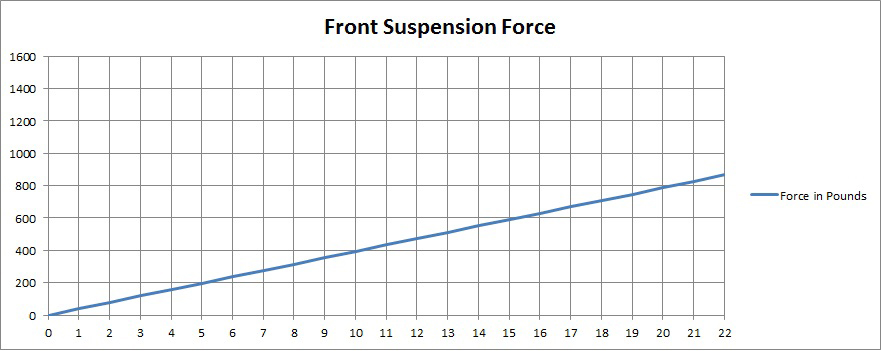Figure 3 is graph that shows the force exerted by a weaker 80 pounds per inch spring. You will note the more gradual slope and lower force than with the 100 pounds per inch spring.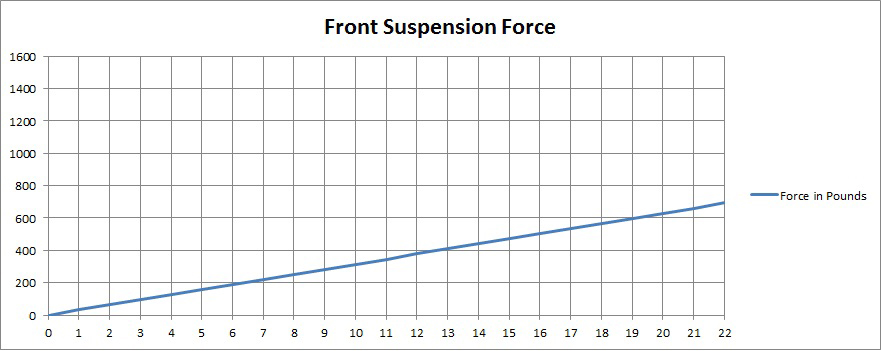This is the second part of the GPL suspension article.

Bump Rubbers:

The bump rubbers are auxillary springs whose main purpose in real life is to prevent the suspension components from hitting and damaging each other when the suspension fully deflects in bump. They are typically made from rubber and only come into play when the suspension is almost fully compressed in bump; hence the name of bump rubber.

In GPL, the bump rubber is treated as a progressive spring and its force is added to the normal spring force.

The bump rubber force is always 800 newtons/179 pounds when fully compressed at the 19 cm suspension deflection point. As players, we cannot adjust the bump rubber's maximum force, but we can adjust the bump rubber length in the setup menu. The bump rubber length is the distance before the full suspension deflection of 19 centimeters at which the bump rubber begins to compress. So if you set a bump rubber length of 2.5 inches/6.35 centimeters, the bump rubber will start to apply force at 19.0-6.35=12.65 centimeters of suspension deflection.

The bump rubber force is progressive so there is very little force added when it first starts to compress. Most of the force comes in the last bit of compression.

Like the springs, the code will extrapolate the bump rubber force so that it continues to increase, but at a linear rate, if the suspension deflection is more than 19 cms.

Figure 4 is a graph that shows the combined force produced by a 100 pounds per inch spring and 2.5 inch bump rubber. You can see that the total suspension force increases at a linear rate solely due to the spring until the 12.65 centimeter point is reached. Then the bump rubber gradually starts adding its force so that at 19 centimeters of suspension deflection, it adds 800 newtons/179 pounds of force. Above 19 cms, the spring and bump rubber forces together increase at a linear rate.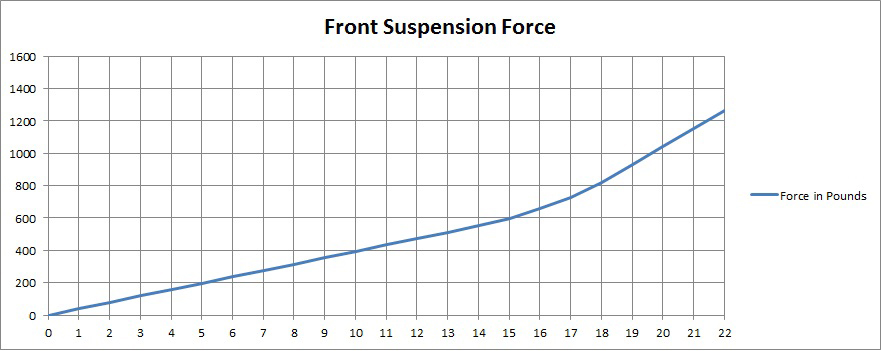Figure 5 is a graph that shows the combined force produced by the same 100 pounds per inch spring and a .5 inch bump rubber. You can see that the bump rubber doesn't come into play until almost the very end of the suspension deflection and its force is added very abruptly.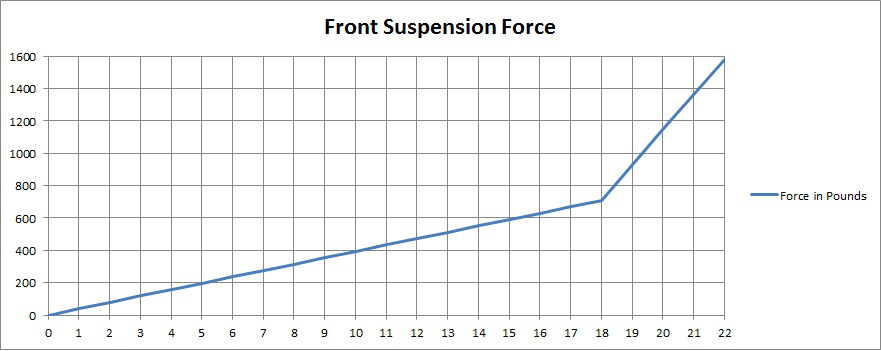So a long bump rubber adds force more gradually than a short bump rubber.

This is the third part of the suspension article.

Static Suspension Deflection:

By definition, the chassis sprung weight sits on the springs. So the suspension deflects and the spring compresses to oppose the weight of the car at rest. The amount of spring compression and the force it produces in return is equal to each wheel's portion of the overall chassis sprung weight.

The amount of sprung weight on each wheel is found by adding up the chassis weight (which includes the oil and water), the fuel weight, and the driver weight and apportioning that total weight by the weight distribution percentage (longitudinal Center of Gravity position as a percentage of the wheelbase).

In GPL, the driver's weight is always 77 KGs. As an example, the '67 Lotus chassis weighs 500 KGs and if we add 30 KGs of fuel, the total sprung weight is (500+30+77=607 KGs/1,335 pounds).

All GPL cars use a weight distribution of about 60% give or take a few percent. So the front wheels will bear 40% of the total sprung weight which is evenly divided between the two front wheels. In our example, the left front wheel will bear (1,335*.40/2=267 pounds).

Now if we were to use a wheel/spring rate of 100 pounds per inch for the left front tire in the setup menu, the static suspension deflection at rest would be (267/100=2.67 inches/6.8 centimeters).

Figure 6 is a graph which shows the total suspension force for a 100 pounds per inch spring and a 2.5 inch bump rubber. The left most red circle is the static suspension deflection point of 6.8 centimeters where the spring is producing exactly 267 pounds of opposing force to the chassis sprung weight.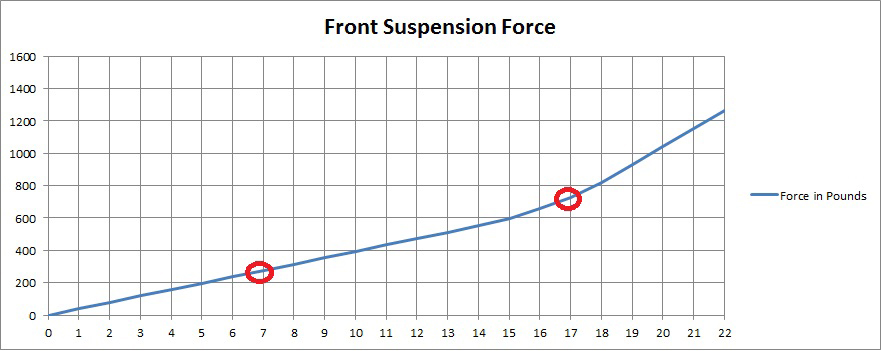Ride Height:

GPL has magic springs. Unlike real world cars where ride height changes with spring strength, GPL first computes the static suspension deflection value and then adds the player setup menu ride height to determine the suspension deflection where the chassis tub bottoms out on the track surface. In other words, it adjusts the spring's attachment point to the chassis so that the desired ride height is achieved when the car is at rest.

Continuing with our example, if we were to use a 4 inch ride height, then 4 inches/10.2 centimeters would be added to the static suspension deflection of 6.8 centimeters. (10.2+6.8=17.0 centimeters). So the suspension deflection would be 17.0 centimeters if the chassis tub bottoms out on landing from a big jump.

With vertical springs and no mechanical advantage, each inch of chassis drop corresponds to one additional inch of suspension deflection/spring compression. So if we use a 4 inch ride height, the suspension deflection/spring compression will increase by 4 inches from static when the chassis tub bottoms.

In Figure 6, the right red circle is the suspension deflection point at which the chassis tub will bottom. As you can see, it occurs when the bump rubber force is active.

Figure 7 is a similar graph that shows the suspension deflection and chassis hub bottom points with the same spring and bump rubbers, but with a 2.5 inch ride height. The static suspension deflection point is the same as before, but the chassis tub bottom point occurs at only 13 centimeters of suspension deflection. This point is just about where the bump rubber begins to add its force. So in effect, the bump rubber is never used.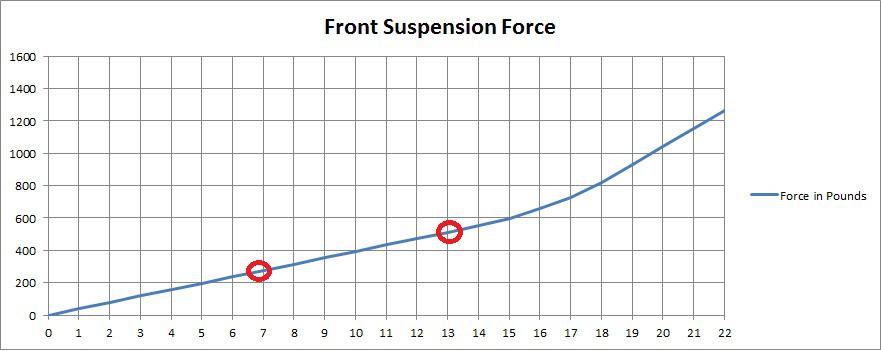This is the fourth and last part of the suspension article.

Implications For Setups:

You now know how GPL's models the suspension as far as the springs, bump rubbers, and ride height are concerned. All three interact to determine the static suspension deflection at rest, the amount of force the suspension produces as you drive around the track and the suspension moves up and down, and the suspension deflection at which the chassis tub will bottom on landing from a jump.

Setups are very personal and there are no right or wrong settings. What works for you may not work for others, but perhaps there are some general rules based on our knowledge of how GPL models the suspension.

Here are some thoughts:

If you look at the total suspension force graph in Figure 4, you can see that there is an initial portion where the spring contributes all the force, then a middle curved portion where the bump rubber force is added, and lastly, the portion where the suspension force continues to increase, but at a linear rate.

I submit that you never want to operate in the far right portion where the suspension force rapidly increases as that may remove any feel for how the car is reacting to suspension movement. So very low wheel/spring rates probably aren't good. Remember that GPL does not model bumps in the track other than artificially so the real world need to use lower wheel/spring rates for improved grip on bumpy tracks does not apply to us.

So that leaves the first two portions of the suspension force curve as our operating area.

You could use a higher wheel/spring rate and .5 inch bump rubber length so that the bump rubbers never come into play before the chassis tub bottoms out. Suspension forces will increase in a linear fashion until the chassis tub bottoms.

Or you could use a lower wheel/spring rate and a 2.5 inch bump rubber so that the chassis tub would bottom just as the maximum suspension deflection of 19 centimeters is reached. This may provide a more gradual increase in suspension forces throughout the suspension's total range and give better feedback to the driver than the first option.

Only tests and your personal preference will tell which option is better for you.